Color

Background

Done

# Faster Searching with Binary Search

by kirupa   |   filed under Data Structures and Algorithms

Earlier, we looked at linear search, a simple algorithm that works by scanning every item in a collection until it finds what it is looking for. While simple is good, this approach is slow. The more items you have, the longer it will take to find something. Its running time is O(n). There is a faster way to search, and that is the star of this article: the binary search.

In the following sections, we will learn all about binary search, how it is implemented, its performance characteristics, and a whole lot more.

Onwards!

## Binary Search in Action

A binary search starts off the same way as many great search algorithms do. It starts with a collection of items: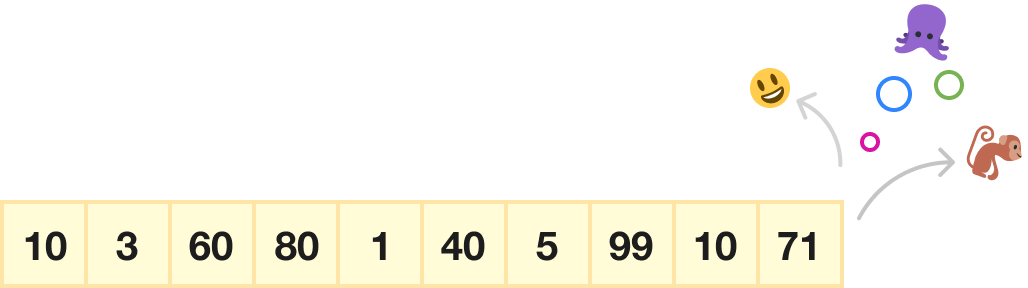In this collection, what we want to do is find the number 60 and return its index position. The rest of our steps will walk through how we can use binary search to accomplish this.

Before we even take our first step, there is an important prerequisite that we need to meet. Our collection of items must already be sorted, so let’s go ahead and do that: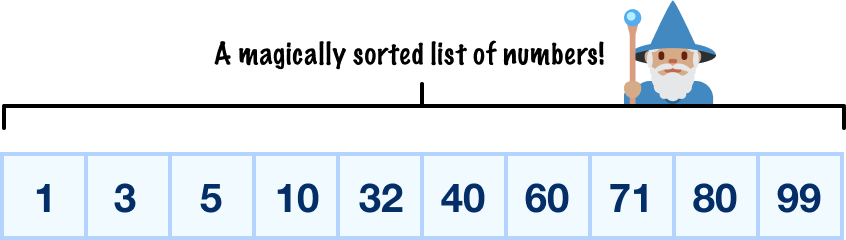In the future, we’ll look into the various ways we have to properly take an unsorted collection and sort the items inside it. For now, let’s just keep it simple and ensure that the items we throw into our binary search algorithm are already sorted.

### Dealing with the Middle Element

With the paperwork out of the way, the first real thing we do is find the middle element of our entire collection and check if the value here is the target we are looking for. For our collection, the middle element is 32: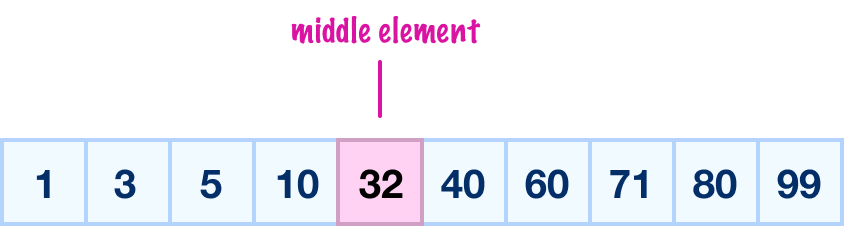How did we get 32? This is especially confusing when we have an even number of items as we do in this example. Let’s talk about this for a brief moment.

When dealing with a collection of odd numbers of elements, we have a clear middle element we can pick: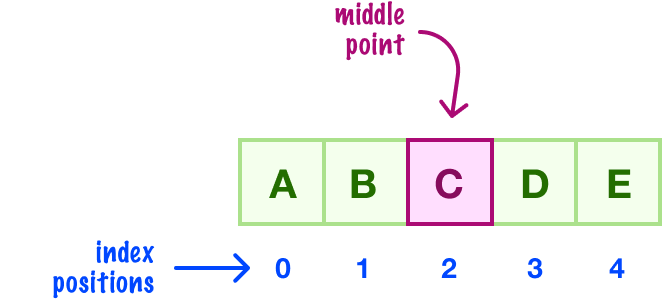As we see here, we have five elements. The third element would be the middle.

For arrays with even numbers of elements, there is a trick we need to use. The middle element will be one that is to the left of the ideal midpoint. This can be visualized as follows: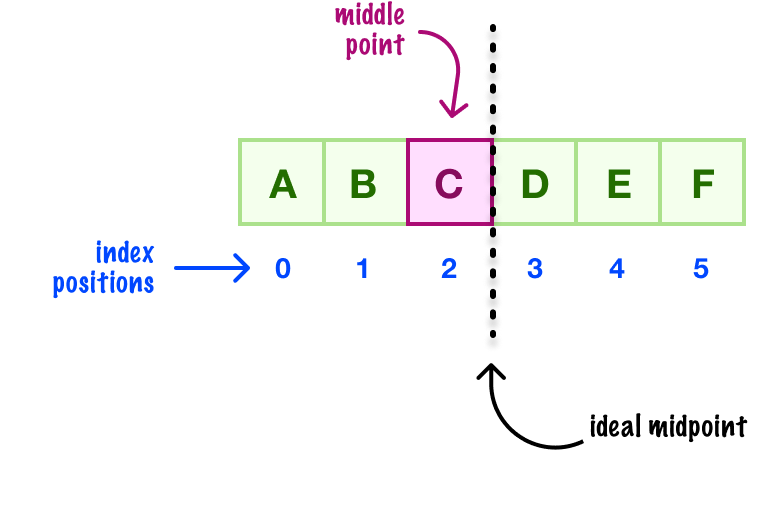We have to do something like this for when we have six elements, like in this example, there is no clear middle element. Either we will be heavy on the left or we will be heavy on the right. There is no way around that, so the general consensus is that we want to lean left towards the start of our collection when we are in a tie-breaking situation. When we apply this trick to our larger example that has an even number of items, we can see how we chose the number 32:Later on, we'll look at a more formal way of finding the middle element that takes our hand-wavy explanation into something more concrete. For now, we are good!

OK, where were we? Yes! We need to check if our middle element contains the target value of 60 that we are looking for. We can tell that 32 is not equal to 60. What we do next is the famed division operation that binary search is known for.

### Dividing FTW!

With our middle element not matching our target value, our next step is to figure out where to go and continue our search. We put our finger on the middle element and mentally divide our list into a left half and a right half: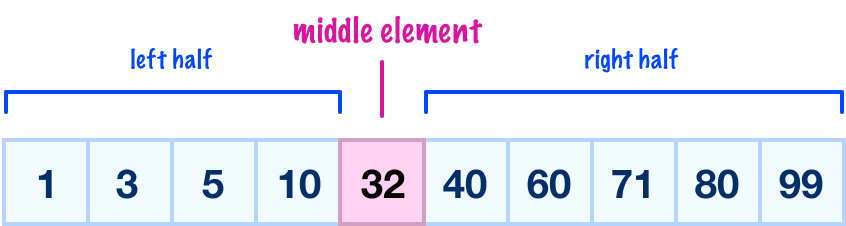For this next step, we ask ourselves whether the value we are looking for (60) is greater than or less than the value in our current middle element (32):

• If the value we are looking for is greater than our middle element value, the value we are looking for must be in the right half
• If the value we are looking for is less than our middle element value, the value we are looking for must be in the left half

In our case, 60 is greater than 32, so it must mean that we need to continue our search by looking in the right half: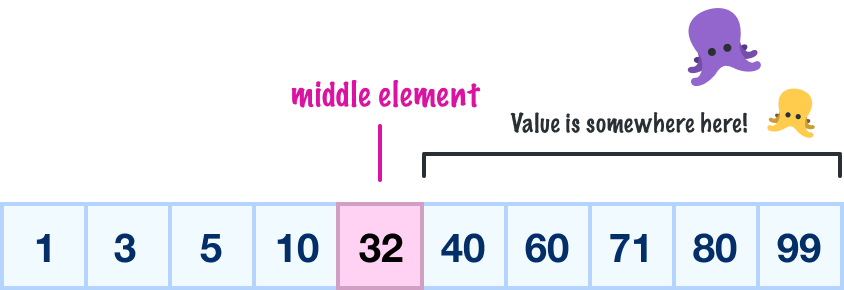At this moment, we only care about what is contained in the right half of our collection. We can safely ignore all other items. To be really specific, this means our earlier midpoint and all items left of it are no longer relevant for our future search activities: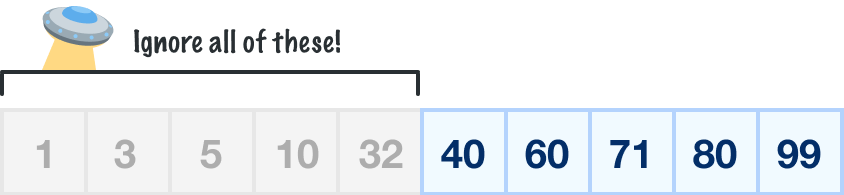With only the right half of our collection in play, we repeat our earlier steps. We find the middle element, check if the middle element’s value matches the target value we are looking for, and if the value doesn’t match, divide and decide whether to go deeper into the remaining left half or right half of our collection.

If we apply these steps to the right half, the first thing we do is find our middle element: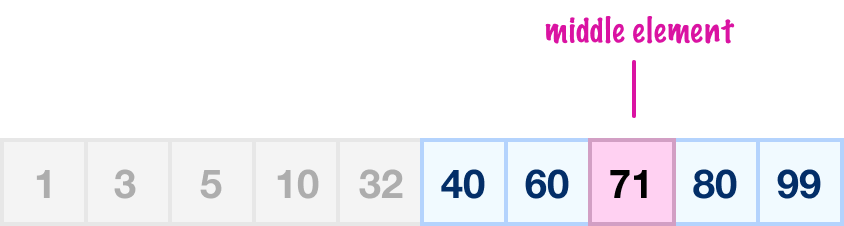The middle element value here is 71, and it isn’t the 60 value we are looking for. Next, we check if 71 is greater than or less than our target 60 value. Because 71 is greater than 60, it means the half of the collection we want to focus on is the left half: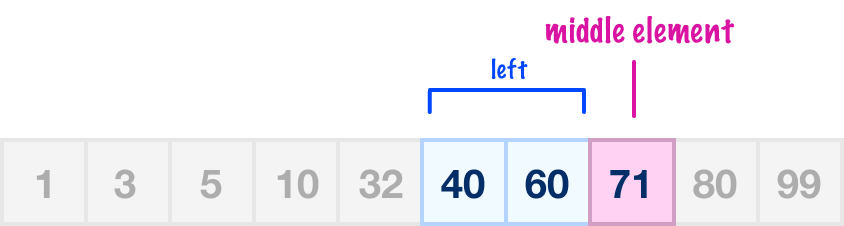When we look at our left half, we only have two items. It doesn’t matter how many items we have, we must still follow our binary search algorithm step-by-step. We must rinse and repeat what we’ve done a few times already. The value of our middle element will be 40: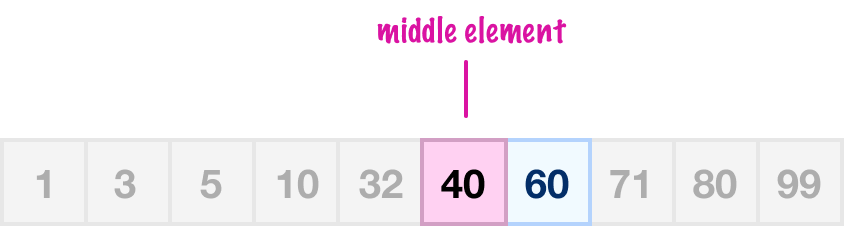Our 40 value is not the same as 60. Because our current middle point value of 40 is less than 60, we focus on the right half: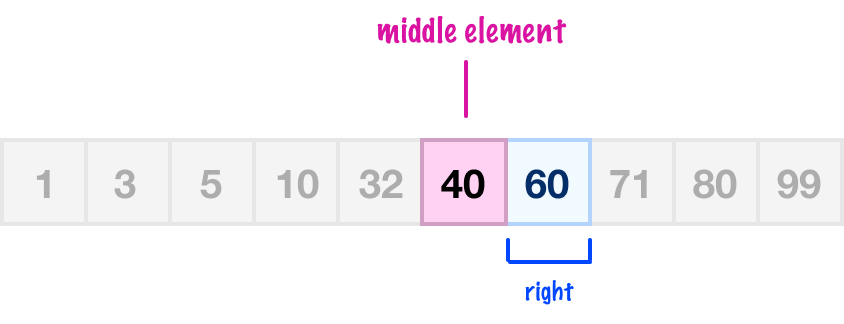We only have one item at this point, and this item will also be our middle element for the next step: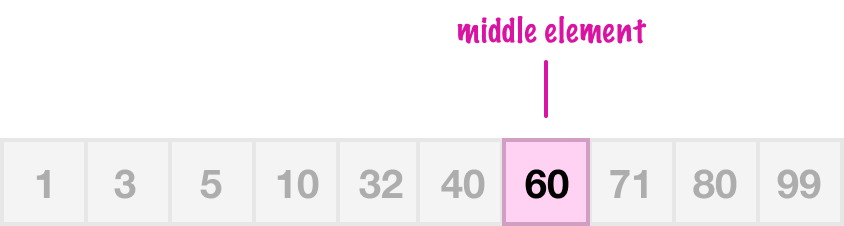The value of our middle element is going to be 60. When we ask ourselves if the middle element value of 60 is what we are looking for, the answer will be a ginormous YES. This is exactly what we have been looking for, and we will return the index position of where our 60 value is located.

## The JavaScript Implementation

If we take all of our many words and visuals from the previous section and simplify how binary search works, we can land on these steps:

1. Look at the middle value in a sorted collection.
2. If our middle value is the target value we are looking for, stop searching and return its index position
1. If it is greater than the value we are looking for, ignore the right half of the collection and repeat step 1 on the left half
2. If it is less than the value we are looking for, ignore the left half of the collection and repeat step 1 on the right half
3. Keep repeating steps 1 to 4 until we find the number we are looking for or determine the value we are looking for is not in the collection

All that remains is to turn these steps into code.

### Iterative Approach

The efficient iterative approach looks as follows:

``````// Iterative Approach
function binarySearch(arr, val) {
let start = 0;
let end = arr.length - 1;

while (start <= right) {
let middleIndex = Math.floor((start + end) / 2);

if (arr[middleIndex] === val) {
return middleIndex;
} else if (arr[middleIndex] < val) {
start = middleIndex + 1;
} else {
end = middleIndex - 1;
}
}

return -1;
}``````

For reasons we saw in the Fibonacci and Going Beyond Recursion article, this approach doesn't inflate the function call stack. We keep a lot of the heavy lifting localized to the loop itself.

### Recursive Approach

For completeness, a less efficient recursive approach is provided below as well:

``````// Recursive Approach
function binarySearch(arr, val, start = 0, end = arr.length - 1) {
const middleIndex = Math.floor((start + end) / 2);

if (val === arr[middleIndex]) {
return middleIndex;
}

if (start >= end) {
return -1;
}

if (val < arr[middleIndex]) {
binarySearch(arr, val, start, middleIndex - 1);
} else {
binarySearch(arr, val, middleIndex + 1, end);
}
}```
```

With this approach, when we are about to do a division, we recursively call binarySearch again. The number of times we will call binarySearch and inflate our call stack is directly related to how deep we will have to search. This is where the efficiency of the iterative approach really shines.

### Example of the Code at Work

The way we would use our binarySearch function is by calling it with two arguments:

1. Our presorted array of items
2. The target value we are looking for

Below is an example of how we can call binarySearch:

``````let numbers = [1, 3, 5, 10, 32, 40, 60, 71, 80, 99];

let foundIndex = binarySearch(numbers, 60);
console.log(foundIndex) // 6``````

In our example, the numbers array is the same collection we spent much of this article deconstructing, so it should look welcomingly familiar. The value that gets returned by our binarySearch function is the index position of the found item or -1 if the item isn't found. In our case, 60 exists and is located at index position 6. Also, outside of the runtime behavior we briefly talked about, the values that get returned are the same across both the iterative and recursive binary search implementations.

#### Finding the Middle Element

Much earlier, we looked at a very hand-wavy approach to finding the middle element. For the full enchilada, this article goes into great detail on this topic. What we are going to do is briefly summarize that earlier article and shine some light on how our code works for finding the middle element, especially as part of a subset of our array.

The expression for calculating the index position of our middle element will look as follows:

``let middleIndex = Math.floor((left + right) / 2);``

We take the average of the starting and ending index positions, and then round the result down (via Math.floor) to ensure we always end on a whole number. If we had to visualize this, here is how we would calculate the middle point for the highlighted region taken from an intermediate step from the walkthrough e we were looking at earlier:The values for left and right are the corresponding 5 and 9 index positions of our array, so if we substitute in those values and calculate the middle point, the earlier expression will look as follows: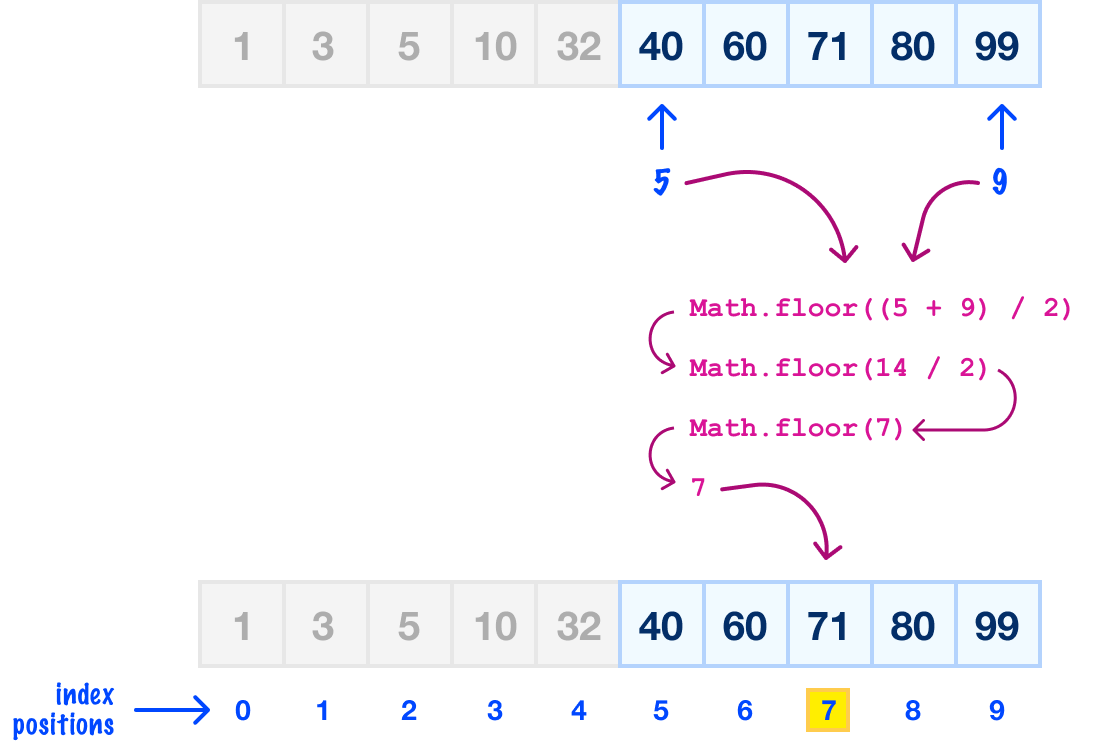Our middle index position for this region is 7, and the value here is 71. A trippy detail to note is that, even though we are only examining a subset of our collection, our index positions are relative to the entire collection itself.

## Runtime Performance

We started off by talking about how fast binary search is, especially when we compare it to the slower linear search. Binary search runs at a scorching O(log n), and it is able to pull this off because it follows a divide and discard approach. As we saw in the walkthrough, at each stage of trying to hone in on our target value, our binary search algorithm ignores half of the remaining items.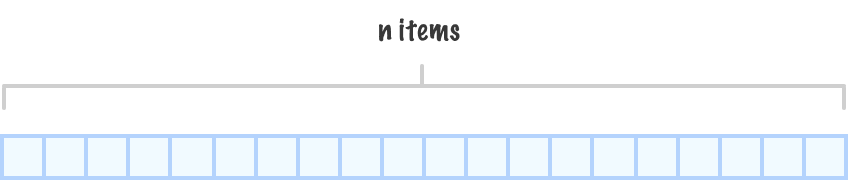In the first step, we will be working with the full n items. At the next step, we will be working with n/2 items:Assuming we never find the value we are looking for (or the value we are looking for is the very last item), this pattern will keep going where each step discards another half of the items from the previous step:We will keep going until we reach the last step, where all we are left with is just a single element. There is a pattern here. We can see this pattern by observing the number of elements in play at each step: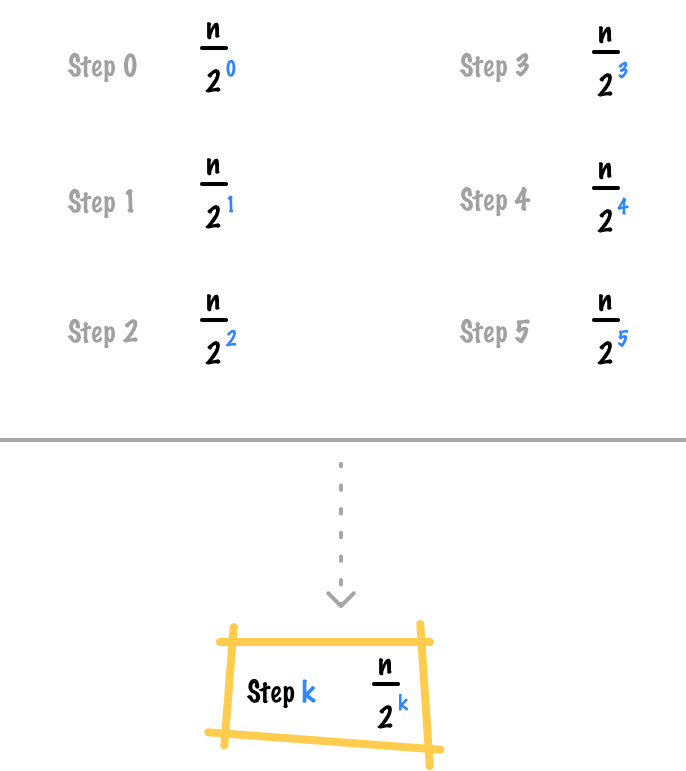If each divide step in our binary search is represented by k, the total number of steps we take can be represented by n / 2k. How does all of this lead to us stating that binary search runs in log(n) time? After k iterations, when we reach the last item in our collection, the number of elements we have left are...well, just 1. This means we can solve for k as follows: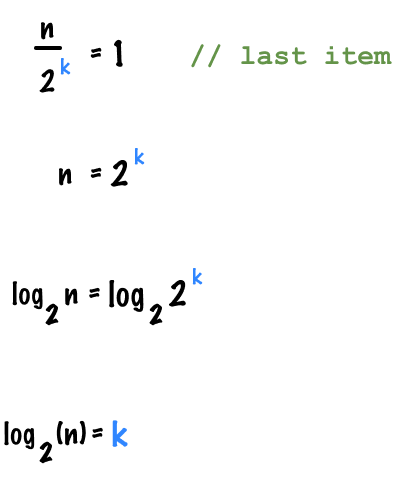The way to read this in the context of binary search is that if we have n items, in the worst case, we need to run through the various stages of our algorithm log(n) times before we reach the end!

## Conclusion

We just covered a lot of ground here. We learned how binary search works, how we can use it in our code, what its runtime behavior looks like, and a whole lot more. What makes binary search interesting is that its core approach of dividing the problem space into something smaller is a common pattern that we'll repeatedly see, especially in algorithms that classify themselves as divide and conquer. We will get to those algorithms shortly, but you should kick back and relax for now. You've earned it!

Just a final word before we wrap up. If you have a question and/or want to be part of a friendly, collaborative community of over 220k other developers like yourself, post on the forums for a quick response!